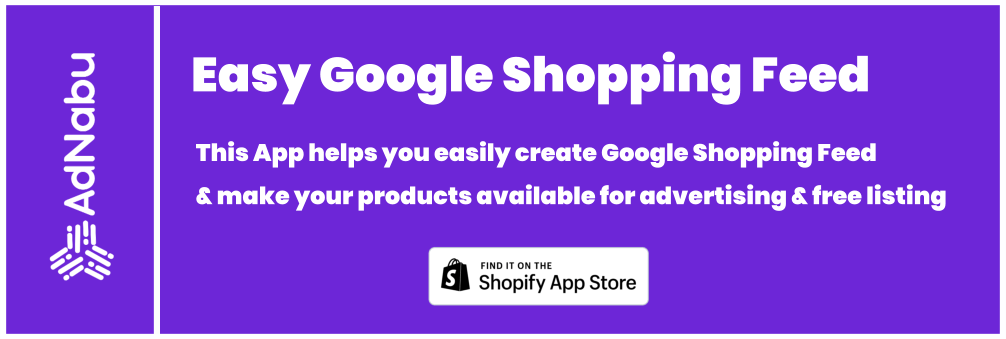As an advertiser lets say you are targeting all relevant keywords from your account and now want to increase your number of conversions per month. The question here is that if it is true that cost-per-conversion increases? And what would the cost come to be?

Most, however, fall into a linear growth model for the cost. But the reality is that cost-per-conversion increases exponentially with the number of conversions.Let us take an example here. An Advertiser generates 1000 conversions a month at 50\$ per conversion resulting in a cost of 50000\$ a month. He wants to generate 1500 conversions a month. To increase the conversion number, the only option is to increase the bids for the targeted keywords. If one increases the bids for keywords, we will generate more clicks at a higher CPC. For a 50% conversion increase, most likely one would need to increase bids by 70% (not a scientific calculation. This number varies with the current position of ads). Since our conversion ratio will not change, this results in 50% increase in conversion number and 70% increase in cost per conversion.

The equation now changes to 1500 conversions a month at 85\$ per conversion which results in a cost of 127500. Here is a summarized result of our exercise.

Scenario Conversions Cost Per Conversion Cost
Before 1000 50 50000
After 1500 85 127500

As you can see from the result, Cost increased by 155% for a 50% increase in conversion. Always keep in mind Cost increases exponentially with Conversions.CEO and co founder of AdNabu. Exploring the intersection of data and marketing